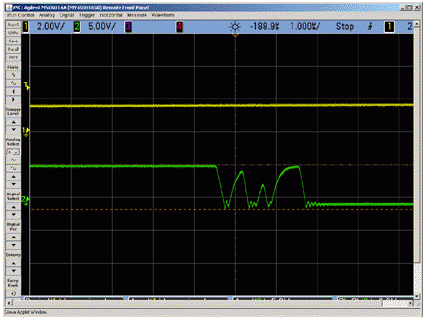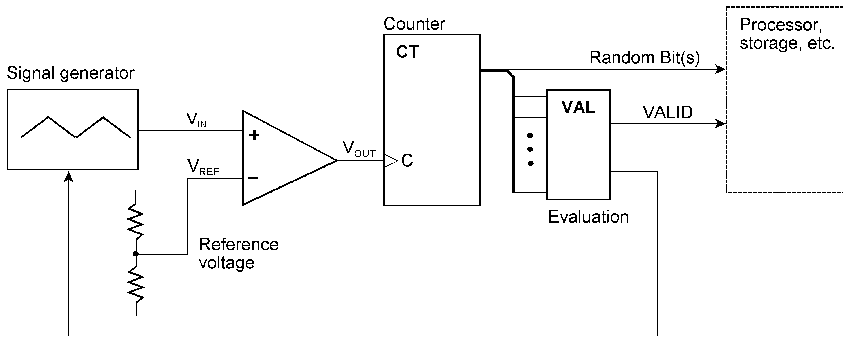Homepage## Microcontrollers —  PCs — Embedded Electronics

True Random Number Generation Using Comparators

 ReAl Computer Projects

This is a long bit string being generated as a true random number (TRN):Those random numbers are called true because they are generated not by algorithms but by physical effects or processes. Various physical phaenomena have been investigated and used to generate true random numbers (TRNs). Here, we will employ the behavior of a well-known electronic component, the comparator.

This is a basic application circuit, a comparator comparing a variable input voltage to a reference voltage.Here the comparator behaves like described in the textbooks. The sinusoidal input voltage is converted into a pulse. Typical comparator ICs have open-collector outputs, where the rise time depends solely on the pull-up resistor and the load capacity (hence the slow rise of the leading edge):The comparator, however, has no built-in snap-action mechanism. Instead, it is simply a high-gain, non-feedback differential amplifier. That the output behaves like a switch results solely from the high voltage gain, causing even a small differential voltage between the two inputs to overdrive the amplifier.

The output level depends on the voltage difference between the inputs. If this difference is positive, the comparator emits a high level. Otherwise, if the difference is negative, the output will assume a low level. This behavior, however, will occur only if the voltage difference is large enough to overdrive the amplifier.

If the voltage difference is very small or zero, no overdriving will occur,  despite the amplifier's large voltage gain. The comparator will behave erratically. Here are two examples showing oscillations caused by a slowly changing input voltage (the yellow trace):Poring over such pictures, the idea occurred that an oscillating comparator could make a  good source of entropy.

The principle is not that complicated. Let the comparator oscillate and count the oscillations. Therefore, we make the input signal slowly traverse the so-called linear region:Here is the experimental platform, built from available modules and components:The microcontroller module generates the input signals, does a first (simplified) evaluation of the count values and supports the communication with an attached personal computer. The prototyping board carries the comparators and a CPLD containing the counters. The human interface module displays raw data and provides for tinkering without the personal computer.

A look at the workplace.  Here with a venerable Tektronix 5441 storage oscilloscope:A closer look at the prototyping board:A typical display on the LCD screen. The numbers are in HEX.Here we show how the random numbers are generated. The yellow trace is the input voltage, generated in small steps by the microntroller's DAC. The green trace shows the comparator's output. The pulses are counted.MORE ...

November 22, 2020

The project's legacy

It's mostly still in German. However, the Google translator should suffice ...

The Xilinx ISE project